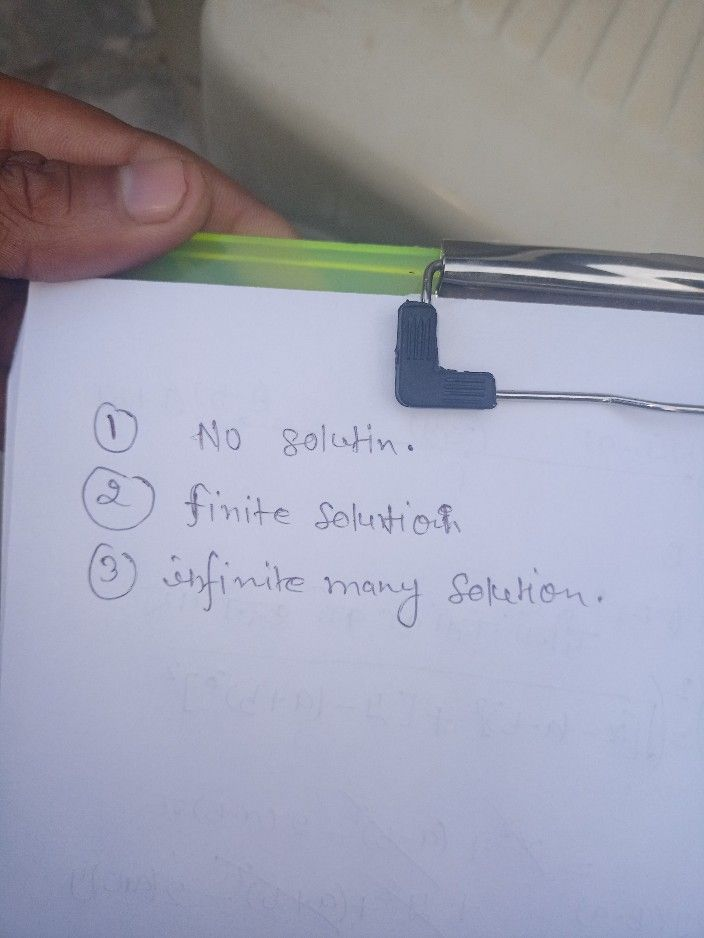Symbol
ProblemLearning Task $1:$ Which of the following graphs has one, non $1n1$ infinite solutions. Write you answer in your notebook. $1$ $2$ $3$ $A$ system of linear equations contains two or more equations e.g.